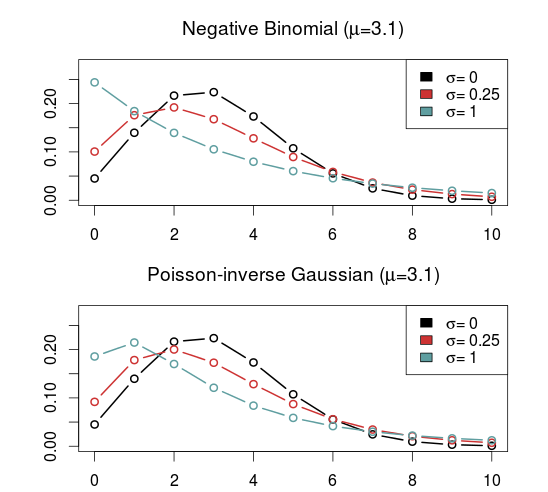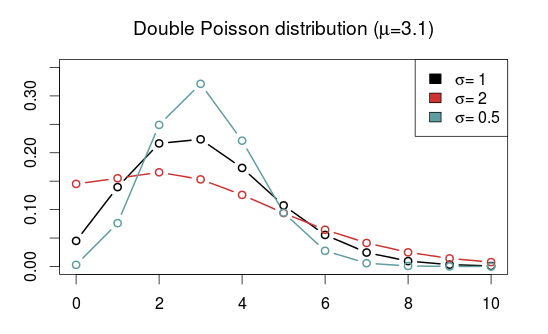# Some alternatives to the Poisson distribution

One important characteristic of the Poisson distribution is that both its expectation and the variance equals parameter $$\lambda$$. A consequence of this is that when we use the Poisson distribution, for example in a Poisson regression, we have to assume that the variance equals the expected value.

The equality assumption may of course not hold in practice and there are two ways in which this assumption can be wrong. Either the variance is less than the expectation or it is greater than the expectation. This is called under- and overdispersion, respectively. When the equality assumption holds, it is called equidispersion.

There are two main consequences if the assumption does not hold: The first is that standard errors of the parameter estimates, which are based on the Poisson, are wrong. This could lead to wrong conclusions when doing inference. The other consequence happens when you use the Poisson to make predictions, for example how many goals a football team will score. The probabilities assigned to each number of goals to be scored will be inaccurate.

(Under- and overdispersion should not be confused with heteroscedasticity in ordinary linear regression. Poisson regression models are naturally heteroscedastic because of the variance-expectation equality. Dispersion refers to what relationship there is between the variance and the expected value, in other words what form the heteroscedasticity takes.)

When it comes to modeling and predicting football results using the Poisson, a good thing would be if the data were actually underdispersed. That would mean that the probabilities for the predicted number of goals scored would be higher around the expectation, and it would be possible to make more precise predictions. The increase in precision would be greatest for the best teams. Even if the data were really overdispersed, we would still get probabilities that more accurately reflect the observed number of goals, although the predictions would be less precise.

This is the reason why I have looked into alternatives to the Poisson model that are suitable to model count data and that are capable of being over- and underdispersed. Except for the negative binomial model there seems to have been little focus on more flexible Poisson-like models in the literature, although there are a handful of papers from the last 15 years with some applied examples.

I should already mention the gamlss package, which is an extremely useful package that can fit a large number of regression type models in R. I like to think of it as the glm function on steroids. It can be used to create regression models for a large number of distributions (50+) and using different forms of dependent variables (for example random effects and splines) and doing regression on distribution parameters other than the usual expectation parameters.

The models that I have considered usually have two parameters. The two parameters are often not easy to interpret, but the distributions can be re-parameterized (which is done in the gamlss package) so that the parameters describe the location (denoted $$\mu$$, often the same as the expectation) and shape (denoted $$\sigma$$, often a dispersion parameter that modifies the association between the expectation and variance). Another typical property is that they equal the Poisson for certain values of the shape parameter.

As I have already mentioned, the kind of model that is most often put forward as an alternative to the Poisson is the Negative binomial distribution (NBI). The advantages of the negative binomial are that is well studied and good software packages exists for using it. The shape parameter $$\sigma > 0$$ determines the overdispersion (relative to the Poisson) so that the closer it is to 0, the more it resembles the Poisson. This is a disadvantage as it can not be used to model underdispersion (or equidispersion, although in practice it can come arbitrarily close to it). Another similar, but less studied, model is the Poisson-inverse Gaussian (PIG). It too has a parameter $$\sigma > 0$$ that determines the overdispersion.A large class of distributions, called Weighted Poisson distributions, is capable of being both over- and underdispersed. (The terms Weighted in the name comes from a technique used to derive the distribution formulas, not that the data is weighted) A paper describing this class can be found here. The general form of the probability distribution is

$$P(x;\theta,\alpha)=\frac{e^{\mu x+\theta t(x)}}{x!C(\theta,\alpha)}$$

where $$t(x)$$ is one of a large number of possible functions, and $$C(\theta,\alpha)$$ is a normalizing constant which makes sure all probabilities in the distribution sum to 1. Note that I have denoted the two parameters using $$\theta$$ and $$\alpha$$ and not $$\mu$$ and $$\sigma$$ to indicate that these are not necessarily location and shape parameters. I think this and interesting class of distributions that I want to look more into, but since they are not generally implemented in any R package that I know of I will not consider them further now.

Another model that is capable of being over- and underdispersed is the Conway–Maxwell–Poisson distribution (COM), which incidentally is a special case of the class of Weighted Poisson distributions mentioned above (see this paper). The Poisson distribution is a special case of the COM when $$\sigma = 1$$, and is underdispersed when $$\sigma > 1$$ and overdispersed when $$\sigma$$ is between 0 and 1. One drawback with the COM model is that the expected value depends on both parameters $$\mu$$ and $$\sigma$$, although it is dominated by $$\mu$$. This makes the interpretation a bit difficult, but it may not be a problem when making predictions.

Unfortunately, the COM model is not supported by the gamlss package, but there are some other R packages that implements it. I have tried a few of them and the only one that I got to work is CompGLM, which for some reason does not use the location ($$\mu$$) and shape ($$\sigma$$) parameterization.The Double Poisson (DP) is another interesting distribution which also equals the Poisson distribution when $$\sigma = 1$$, but is overdispersed when $$\sigma > 1$$ and underdispersed when $$\sigma$$ is between 0 and 1. The expectation does not depend on the shape parameter $$\sigma$$, and it is approximately equal to the location parameter $$\mu$$. Another interesting thing about the Double Poisson is that it is belongs to a larger group of distributions called double exponential families which also lets you derive a binomial-like distribution with an extra dispersion parameter which can be useful in a logistic regression setting (see this paper, or this preprint).In a follow up post I will try to use these distributions in regression models similar to the independent Poisson model.

# Predicting football results with Poisson regression pt. 1

I have been meaning to write about my take on using Poisson regression to predict football results for a while, so here we go. Poisson regression is one of the earliest statistical methods used for predicting football results. The goal here is to use available data to to say something about how many goals a team is expected to score and from that calculate the probabilities for different match outcomes.

The Poisson distribution
The Poisson distribution is a probability distribution that can be used to model data that can be counted (i.e something that can happen 0, 1, 2, 3, … times). If we know the number of times something is expected to happen, we can find the probabilities that it happens any number of times. For example if we know something is expected to happen 4 times, we can calculate the probabilities that it happens 0, 1, 2, … times.

It turns out that the number of goals a team scores in a football match are approximately Poisson distributed. This means we have a method of assigning probabilities to the number of goals in a match and from this we can find probabilities for different match results. Note that I write that goals are approximately Poisson. The Poisson distribution does not always perfectly describe the number of goals in a match. It sometimes over or under estimates the number of goals, and some football leagues seems fit the Poisson distribution better than others. Anyway, the Poisson distribution seems to be an OK approximation.

The regression model
To be able to find the probabilities for different number of goals we need to find the expected number of goals L (It is customary to denote the expectation in a Poisson distribution by the Greek letter lambda, but WordPress seem to have problems with greek letters so i call i L instead). This is where the regression method comes in. With regression we can estimate lambda conditioned on certain variables. The most obvious variable to look at is which team is playing. Manchester United obviously makes more goals than Wigan. The second thing we want to take into account is who the opponent is. Some teams are expected to concede fewer goals, while others are expected to let in more goals. The third thing we want to take into account is home field advantage.

Written in the language of regression models this becomes

log(L) = mu + home + teami + opponentj

The mu is the overall mean number of goals. The home is the effect on number of goals a team has by playing at home. Teami is the effect of team number i, opponentj is the effect of team j.

(Note: Some descriptions of the Poisson regression model on football data uses the terms offensive and defensive strength to describe what I have called team and opponent. The reason I prefer the terms I use here is because it makes it a bit easier to understand later when we look at the data set.)

The logarithm on the left hand side is called the link function. I will not dwell much on what a link function is, but the short story is that they ensure that the parameter we try to estimate don’t fall outside its domain. In this case it ensures us that we never get negative expected number of goals.

Data
In my example I will use data from football-data.co.uk. What data you would want to use is up to yourself. Typically you could choose to use data from the last year or the least season, but that is totally up to you to decide.

Each of the terms on the right hand side of the equation (except for mu) corresponds to a columns in a table, so we need to fix our data a bit before we proceed with fitting the model. Each match is essentially two observations, one for how many goals the home team scores, the second how many the away team scores. Basically, each match need two rows in our data set, not just one.

Doing the fix is an easy thing to do in excel or Libre Office Calc. We take the data rows (i.e. the matches) we want to use and duplicate them. Then we need to switch the away team and away goals columns so they become the same as the home team column. We also need a column to indicate the home team. Here is an example on how it will look like:In the next part I will fit the actual model, calculate probabilities and describe how we can make predictions using R.